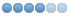# How to increase stats without having to remove gear or rent

• Nero
•Topic Author
• Offline
•1 month 2 days ago - 1 month 2 days ago #10255 by Nero
I have an annoying situation where I have a training hall players can go to to increase their stats (str, int, wis, dex, con, cha)
Everything works but one issue I am seeing is that if a player increases their con for example, it will show that their maximum abilities went up
but the modified abilities (which is what it should be with gear, buffs, etc) stays at the same. I have to remove my gear and put it back on OR rent out and come back in for the increase to the modified abilities to show the change and update my hitpoints.
Informative.c
``` send_to_char(ch, "@yMaximum Abilities:@n  Str[%2d]  Int[%2d]  Wis[%2d]  Dex[%2d]  Con[%2d]  Char[%2d]\r\n",
GET_STAT_MAX(ch, ABILITY_STR), GET_STAT_MAX(ch, ABILITY_INT),
GET_STAT_MAX(ch, ABILITY_WIS), GET_STAT_MAX(ch, ABILITY_DEX),
GET_STAT_MAX(ch, ABILITY_CON), GET_STAT_MAX(ch, ABILITY_CHA));
send_to_char(ch, "@yModified Abilities:@n Str[%2d]  Int[%2d]  Wis[%2d]  Dex[%2d]  Con[%2d]  Char[%2d]\r\n",
GET_TOT_STR(ch), GET_TOT_INT(ch), GET_TOT_WIS(ch), GET_TOT_DEX(ch),
GET_TOT_CON(ch), GET_TOT_CHA(ch));
send_to_char(ch, "@yNatural Abilities:@n  Str[%2d]  Int[%2d]  Wis[%2d]  Dex[%2d]  Con[%2d]  Char[%2d]\r\n",
GET_NAT_STR(ch), GET_NAT_INT(ch), GET_NAT_WIS(ch), GET_NAT_DEX(ch),
GET_NAT_CON(ch), GET_NAT_CHA(ch));```

/* Racial automatic affects */ This is in Handler.c
/* These effects were being removed when items with the same effects were removed. */
/* Jason M. Phillips 9/28/2020 */
if (IS_TRITON(ch)) SET_BIT_AR(AFF_FLAGS(ch), AFF_WATERBREATH);
if (IS_TRITON(ch)) SET_BIT_AR(AFF_FLAGS(ch), AFF_WATERWALK);
if (IS_HALFLING(ch)) SET_BIT_AR(AFF_FLAGS(ch), AFF_INFRAVISION);
if (IS_PIXIE(ch)) SET_BIT_AR(AFF_FLAGS(ch), AFF_AIRWALK);
if (IS_WEMIC(ch)) SET_BIT_AR(AFF_FLAGS(ch), AFF_INFRAVISION);

/* NPC and PC Str, Int, Wis, Dex, Con, Cha adjustments */
if (IS_NPC(ch)) {
GET_TOT_STR(ch) = MAX(0, MIN(GET_NAT_STR(ch) + GET_MOD_STR(ch), MAX_STAT_ATTRIBUTE));
GET_TOT_INT(ch) = MAX(0, MIN(GET_NAT_INT(ch) + GET_MOD_INT(ch), MAX_STAT_ATTRIBUTE));
GET_TOT_WIS(ch) = MAX(0, MIN(GET_NAT_WIS(ch) + GET_MOD_WIS(ch), MAX_STAT_ATTRIBUTE));
GET_TOT_DEX(ch) = MAX(0, MIN(GET_NAT_DEX(ch) + GET_MOD_DEX(ch), MAX_STAT_ATTRIBUTE));
GET_TOT_CON(ch) = MAX(0, MIN(GET_NAT_CON(ch) + GET_MOD_CON(ch), MAX_STAT_ATTRIBUTE));
GET_TOT_CHA(ch) = MAX(0, MIN(GET_NAT_CHA(ch) + GET_MOD_CHA(ch), MAX_STAT_ATTRIBUTE));
}
else {
GET_TOT_STR(ch) = MAX(0, MIN(GET_NAT_STR(ch) + GET_MOD_STR(ch), GET_STAT_MAX(ch, ABILITY_STR)));
GET_TOT_INT(ch) = MAX(0, MIN(GET_NAT_INT(ch) + GET_MOD_INT(ch), GET_STAT_MAX(ch, ABILITY_INT)));
GET_TOT_WIS(ch) = MAX(0, MIN(GET_NAT_WIS(ch) + GET_MOD_WIS(ch), GET_STAT_MAX(ch, ABILITY_WIS)));
GET_TOT_DEX(ch) = MAX(0, MIN(GET_NAT_DEX(ch) + GET_MOD_DEX(ch), GET_STAT_MAX(ch, ABILITY_DEX)));
GET_TOT_CON(ch) = MAX(0, MIN(GET_NAT_CON(ch) + GET_MOD_CON(ch), GET_STAT_MAX(ch, ABILITY_CON)));
GET_TOT_CHA(ch) = MAX(0, MIN(GET_NAT_CHA(ch) + GET_MOD_CHA(ch), GET_STAT_MAX(ch, ABILITY_CHA)));
}